# Manacher’s Algorithm – Linear Time Longest Palindromic Substring – Part 1

• Difficulty Level : Hard
• Last Updated : 11 Feb, 2021

Given a string, find the longest substring which is palindrome.

• if the given string is “forgeeksskeegfor”, the output should be “geeksskeeg”
• if the given string is “abaaba”, the output should be “abaaba”
• if the given string is “abababa”, the output should be “abababa”
• if the given string is “abcbabcbabcba”, the output should be “abcbabcbabcba”

We have already discussed Naïve [O(n3)] and quadratic [O(n2)] approaches at Set 1 and Set 2
In this article, we will talk about Manacher’s algorithm which finds Longest Palindromic Substring in linear time.
One way (Set 2) to find a palindrome is to start from the center of the string and compare characters in both directions one by one. If corresponding characters on both sides (left and right of the center) match, then they will make a palindrome.
Let’s consider string “abababa”.
Here center of the string is 4th character (with index 3) b. If we match characters in left and right of the center, all characters match and so string “abababa” is a palindrome.Here center position is not only the actual string character position but it could be the position between two characters also.
Consider string “abaaba” of even length. This string is palindrome around the position between 3rd and 4th characters a and a respectively.To find Longest Palindromic Substring of a string of length N, one way is take each possible 2*N + 1 centers (the N character positions, N-1 between two character positions and 2 positions at left and right ends), do the character match in both left and right directions at each 2*N+ 1 centers and keep track of LPS. This approach takes O(N^2) time and that’s what we are doing in Set 2

Let’s consider two strings “abababa” and “abaaba” as shown below: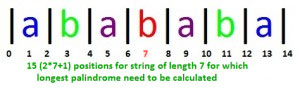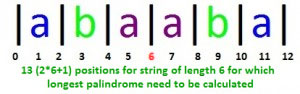In these two strings, left and right side of the center positions (position 7 in 1st string and position 6 in 2nd string) are symmetric. Why? Because the whole string is palindrome around the center position.
If we need to calculate Longest Palindromic Substring at each 2*N+1 positions from left to right, then palindrome’s symmetric property could help to avoid some of the unnecessary computations (i.e. character comparison). If there is a palindrome of some length L centered at any position P, then we may not need to compare all characters in left and right side at position P+1. We already calculated LPS at positions before P and they can help to avoid some of the comparisons after position P.
This use of information from previous positions at a later point of time makes the Manacher’s algorithm linear. In Set 2, there is no reuse of previous information and so that is quadratic.

Manacher’s algorithm is probably considered complex to understand, so here we will discuss it in as detailed way as we can. Some of it’s portions may require multiple reading to understand it properly.

Let’s look at string “abababa”. In 3rd figure above, 15 center positions are shown. We need to calculate length of longest palindromic string at each of these positions.

• At position 0, there is no LPS at all (no character on left side to compare), so length of LPS will be 0.
• At position 1, LPS is a, so length of LPS will be 1.
• At position 2, there is no LPS at all (left and right characters a and b don’t match), so length of LPS will be 0.
• At position 3, LPS is aba, so length of LPS will be 3.
• At position 4, there is no LPS at all (left and right characters b and a don’t match), so length of LPS will be 0.
• At position 5, LPS is ababa, so length of LPS will be 5.

…… and so on

We store all these palindromic lengths in an array, say L. Then string S and

Length L look like below: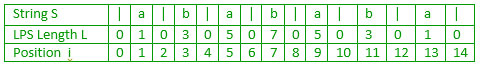Similarly, LPS Length L of string “abaaba” will look like: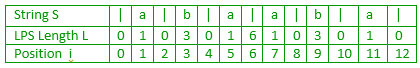In LPS Array L:

• LPS length value at odd positions (the actual character positions) will be odd and greater than or equal to 1 (1 will come from the center character itself if nothing else matches in left and right side of it)
• LPS length value at even positions (the positions between two characters, extreme left and right positions) will be even and greater than or equal to 0 (0 will come when there is no match in left and right side)

Position and index for the string are two different things here. For a given string S of length N, indexes will be from 0 to N-1 (total N indexes) and positions will be from 0 to 2*N (total 2*N+1 positions).

LPS length value can be interpreted in two ways, one in terms of index and second in terms of position. LPS value d at position I (L[i] = d) tells that:

• Substring from position i-d to i+d is a palindrome of length d (in terms of position)
• Substring from index (i-d)/2 to [(i+d)/2 – 1] is a palindrome of length d (in terms of index)

e.g. in string “abaaba”, L = 3 means substring from position 0 (3-3) to 6 (3+3) is a palindrome which is “aba” of length 3, it also means that substring from index 0 [(3-3)/2] to 2 [(3+3)/2 – 1] is a palindrome which is “aba” of length 3.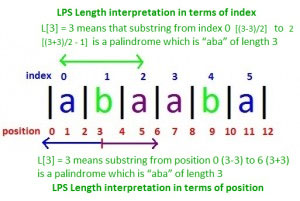Now the main task is to compute LPS array efficiently. Once this array is computed, LPS of string S will be centered at position with maximum LPS length value.
We will see it in Part 2